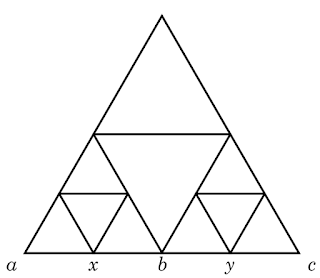Ir al contenido principal

## Due May 24th

### Problem 1

The minimum of

$f(x,y,z) =$
$(a-x)^2 + (x-y)^2 + (y-a)^2 + (x-b)^2 + (b-z)^2 + (z-x)^2 + (y-z)^2 + (z-c)^2 + (c-y)^2$
is attained at
$\displaystyle x^* = \frac{2a+2b+c}{5},\; y^* = \frac{2a+b+2c}{5},\; z^* = \frac{a+2b+2c}{5},$
with

### Problem 2

One can obtain the values $u(f_2(q_1)) = x, u(f_3(q_1)) = y$ of a harmonic function in terms of the values $a,b,c$ at the points $p_2, q_1, p_3$, respectively  (as in the figure below).### Problem 3

If $u$ is a harmonic function with boundary values $u(p_1) = u(p_2) = 0$ and $u(p_3) = 1$, then its restriction to the bottom side of the Sierpinski triangle is an increasing function on $[0,1]$. (Hint: Use the previous problem.)

### Problem 4

If $u$ is harmonic on the Sierpinski gasket $S$, then there exists a constant $c>0$ such that
$|u(x) - u(y)| \le c \Big(\dfrac{3}{5}\Big)^m$
if $x\sim y$ in $V_m$.

### Problem 5

If $u$ is harmonic on the Sierpinski gasket $S$, then it is uniformly continuous on $V_*$ and thus extends to a continuous function on $S$.